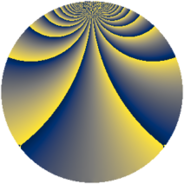# Properties

 Label 1210.2.lLevel $1210$ Weight $2$ Character orbit 1210.l Rep. character $\chi_{1210}(233,\cdot)$ Character field $\Q(\zeta_{20})$ Dimension $432$ Sturm bound $396$

# Related objects

## Defining parameters

 Level: $$N$$ $$=$$ $$1210 = 2 \cdot 5 \cdot 11^{2}$$ Weight: $$k$$ $$=$$ $$2$$ Character orbit: $$[\chi]$$ $$=$$ 1210.l (of order $$20$$ and degree $$8$$) Character conductor: $$\operatorname{cond}(\chi)$$ $$=$$ $$55$$ Character field: $$\Q(\zeta_{20})$$ Sturm bound: $$396$$

## Dimensions

The following table gives the dimensions of various subspaces of $$M_{2}(1210, [\chi])$$.

Total New Old
Modular forms 1776 432 1344
Cusp forms 1392 432 960
Eisenstein series 384 0 384

## Trace form

 $$432q + 8q^{3} + 8q^{5} + 20q^{7} + O(q^{10})$$ $$432q + 8q^{3} + 8q^{5} + 20q^{7} + 32q^{12} + 20q^{15} + 108q^{16} + 20q^{17} + 8q^{20} + 24q^{23} + 8q^{25} - 8q^{26} - 16q^{27} + 20q^{28} - 16q^{31} + 124q^{36} - 32q^{37} + 36q^{38} + 20q^{41} + 20q^{42} - 64q^{45} - 40q^{46} - 36q^{47} - 8q^{48} - 40q^{50} - 40q^{51} - 40q^{52} - 4q^{53} + 8q^{56} - 48q^{58} - 16q^{60} - 80q^{61} - 40q^{62} - 100q^{63} + 16q^{67} - 20q^{68} + 72q^{70} + 96q^{71} + 20q^{73} + 88q^{75} - 16q^{78} + 12q^{80} + 228q^{81} + 16q^{82} + 80q^{85} + 56q^{86} + 80q^{90} + 68q^{91} + 16q^{92} - 76q^{93} - 20q^{95} - 84q^{97} + O(q^{100})$$

## Decomposition of $$S_{2}^{\mathrm{new}}(1210, [\chi])$$ into newform subspaces

The newforms in this space have not yet been added to the LMFDB.

## Decomposition of $$S_{2}^{\mathrm{old}}(1210, [\chi])$$ into lower level spaces

$$S_{2}^{\mathrm{old}}(1210, [\chi]) \cong$$ $$S_{2}^{\mathrm{new}}(55, [\chi])$$$$^{\oplus 4}$$$$\oplus$$$$S_{2}^{\mathrm{new}}(110, [\chi])$$$$^{\oplus 2}$$$$\oplus$$$$S_{2}^{\mathrm{new}}(605, [\chi])$$$$^{\oplus 2}$$